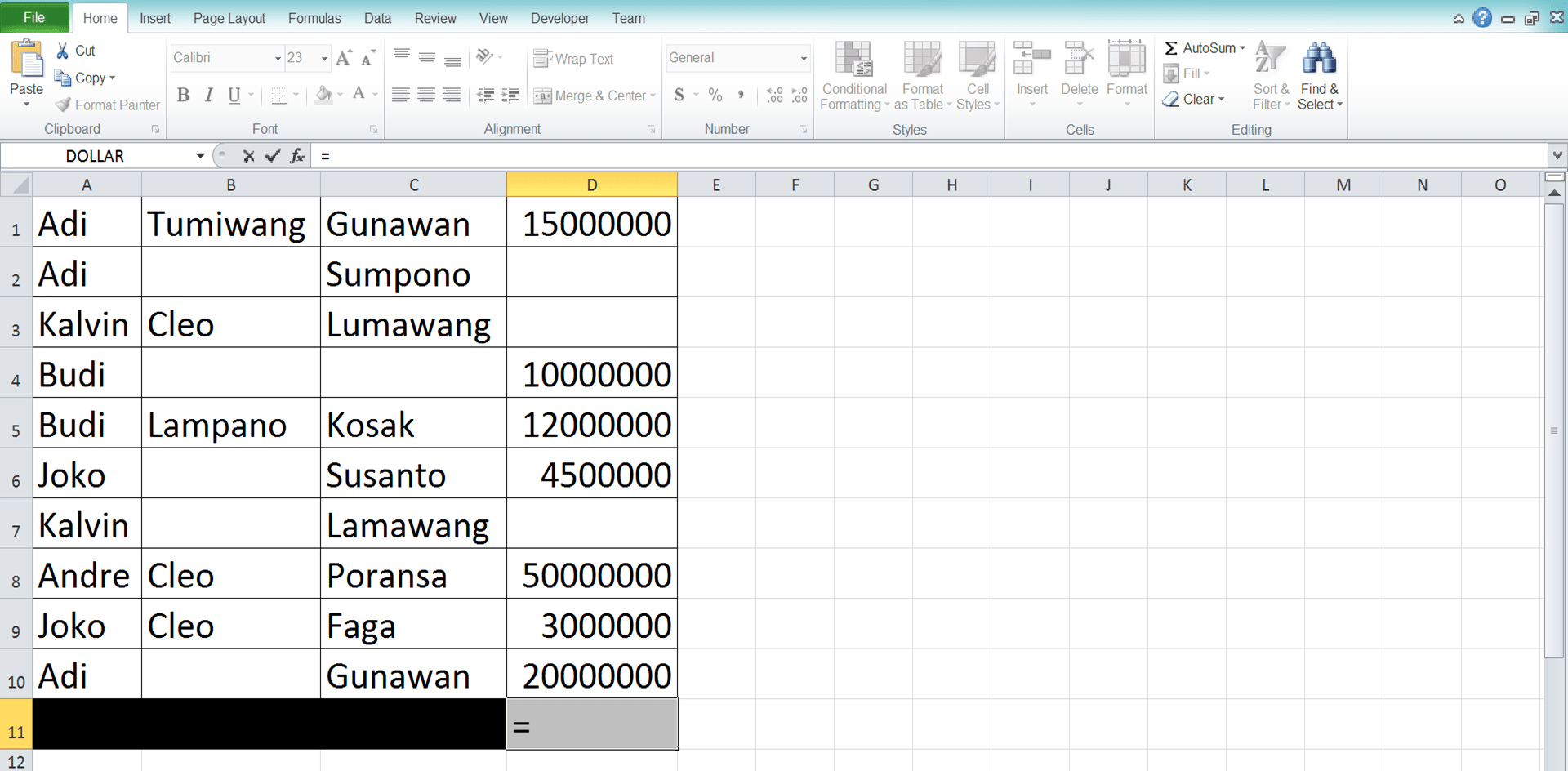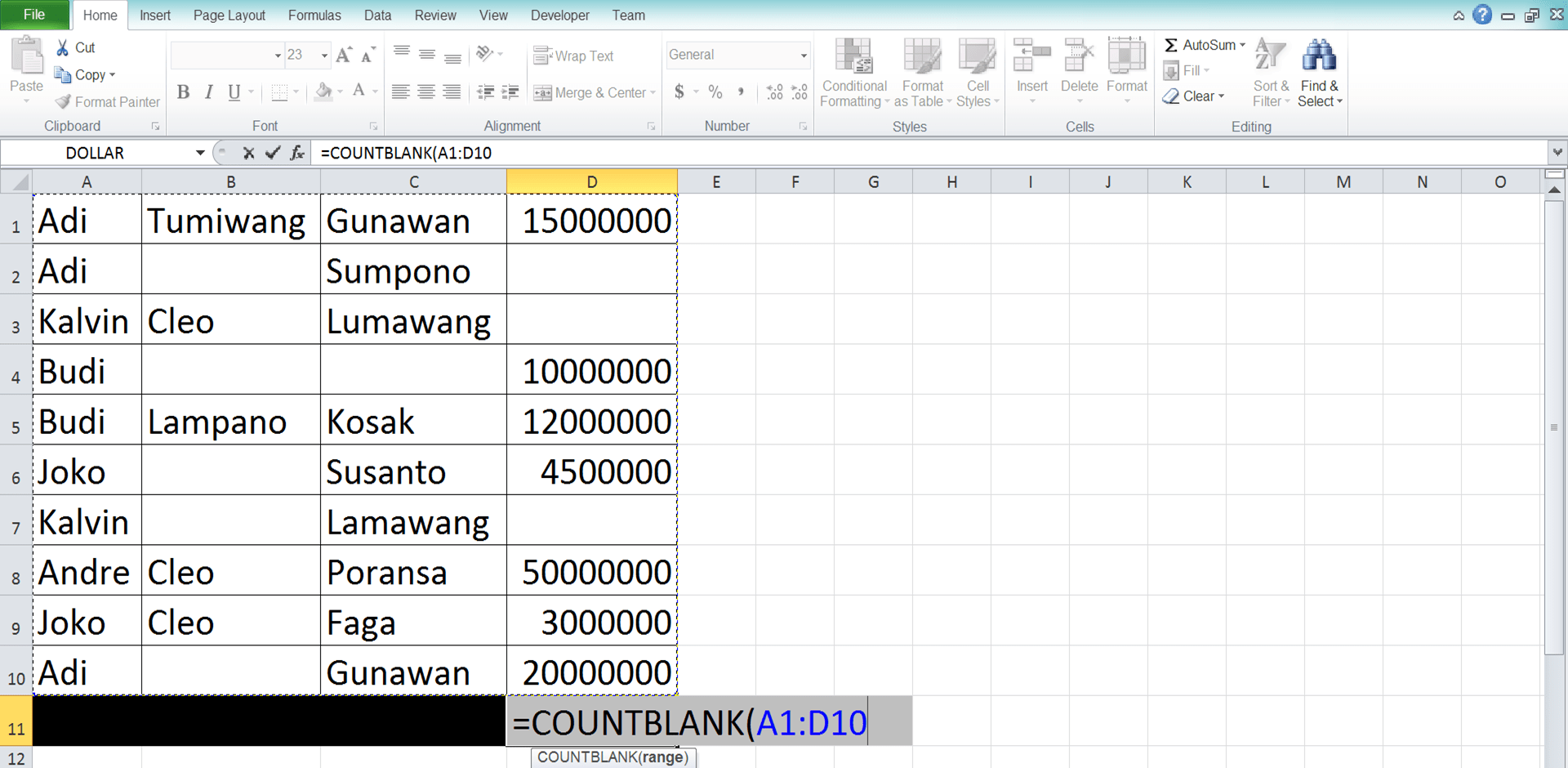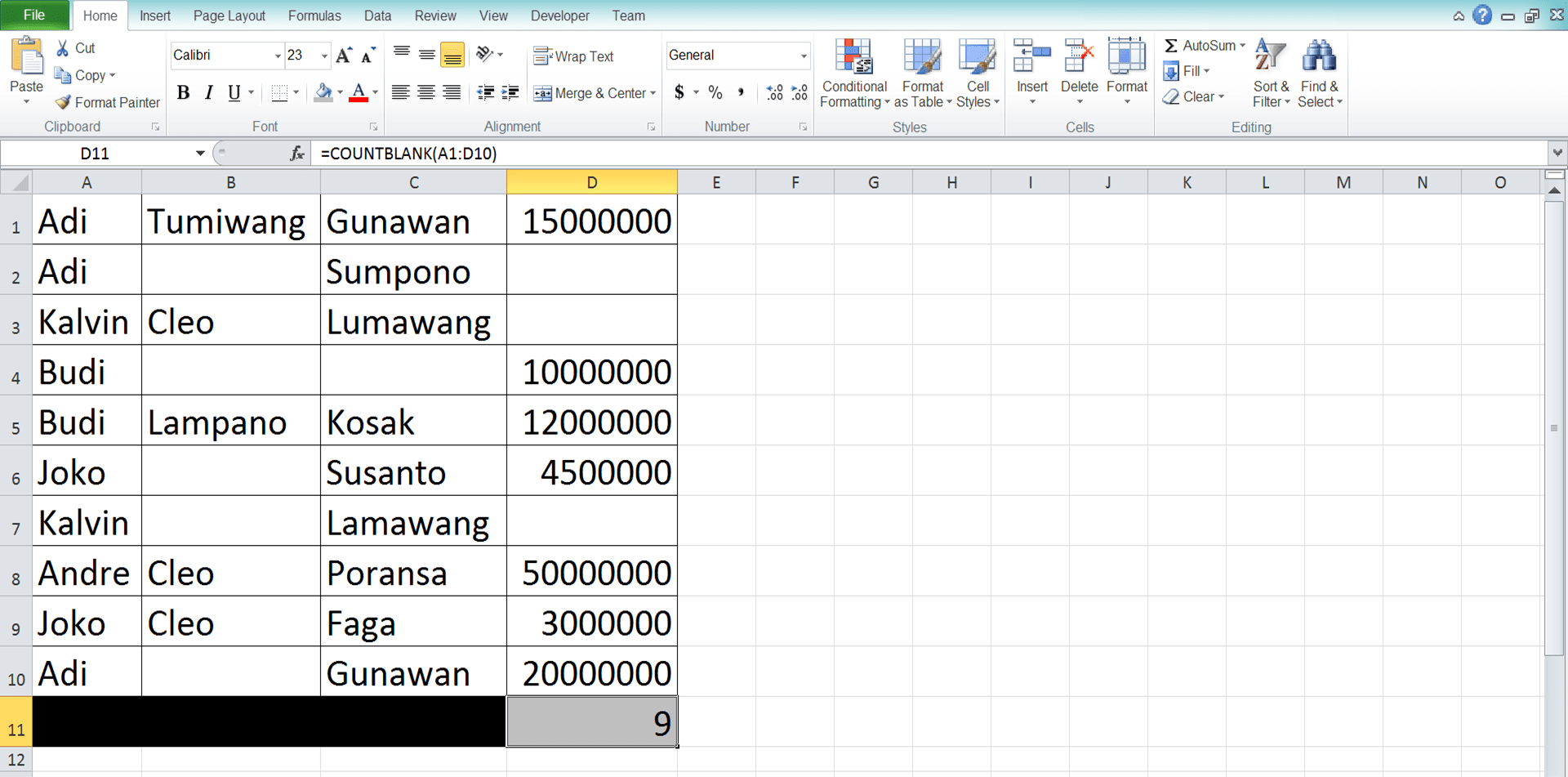How to Use the COUNTBLANK Function in Excel: Usabilities, Examples, and Its Writing Steps - Compute Expert

# How to Use the COUNTBLANK Function in Excel: Usabilities, Examples, and Its Writing Steps

Home >> Excel Tutorials from Compute Expert >> Excel Formulas List >> How to Use the COUNTBLANK Function in Excel: Usabilities, Examples, and Its Writing Steps

In this tutorial, you will learn how to use the COUNTBLANK function in excel completely.

When working in excel, we sometimes need to know how many blank cells do we have in a cell range. COUNTBLANK can help you to get that kind of information fast.

Want to understand more about the way to use this formula in excel? Read this tutorial until its last part!

Disclaimer: This post may contain affiliate links from which we earn commission from qualifying purchases/actions at no additional cost for you. Learn more

## What is the COUNTBLANK Function in Excel?

COUNTBLANK function is an excel function you can use to count the number of blank cells in a cell range. Blank cells here mean the cells contain empty data. That includes cells that contain empty text (we usually represent empty text with empty double quotes ( “” )).

## COUNTBLANK Usability

You can use COUNTBLANK to get the number of blanks you have in a specific cell range.

## COUNTBLANK Result

The COUNTBLANK result is a number that represents the number of blank cells in the cell range you input into COUNTBLANK.

## Excel Version from Which We Can Start Using COUNTBLANK

We can start using COUNTBLANK since excel 2003.

## The Way to Write It and Its Input

Here is the general writing form of a COUNTBLANK formula in excel.

= COUNTBLANK ( cell_range )

The only input you give to COUNTBLANK is the cell range you want to count the number of blank cells of.

Unlike other count formulas in excel (COUNT, COUNTA, etc), you can only input one cell range to COUNTBLANK.

## Example of Its Usage and Result

Here is the implementation example of COUNTBLANK in excel.As you can see in the example, we only need to input the cell range we want to process to COUNTBLANK. As the blank cells in the cell range input here are two, that is the number that COUNTBLANK returns to us.

## Writing Steps

After we have discussed the COUNTBLANK writing form, input, and implementation example, now let’s discuss its writing steps. As the input COUNTBLANK needs is only one cell range, you should be able to understand its writing steps quite easily!

1. Type equal sign ( = ) in the cell where you want to put your COUNTBLANK result2. Type COUNTBLANK (can be with large and small letters) and an open bracket sign after =3. Input the cell range you want to count the number of blank cells from4. Type a close bracket sign5. Press Enter
6. Done!## Exercise

After you have learned how to use the COUNTBLANK formula in excel, now let’s do an exercise. This is so you can practice the lessons you have got from this tutorial!

### Questions

Use COUNTBLANK to answer each of the questions below!
1. How many blank cells are there in the product name column?
2. How many blank cells are there in the stock quantities and today’s sales columns?
3. How many blank cells are there in the product name, stock quantities, and today’s sales column?

COUNTBLANK won’t count zero numbers, spaces, or errors in the cell range you input into it. It will only count empty cells and cells with empty data (cells that contain empty quotes).

Related tutorials you should learn from:

Get updated excel info from Compute Expert by registering your email. It's free!